# 6+ Best Flow Measurement Techniques used in Industries

Hey Friends! Welcome to another article on Instrumentationblog.com!

Today in this article, we are going to see the fundamentals of Flow Measurement and how the flow can be calculated via the PLC program.

# Flow Measurement

Flow measurement can be defined as the quantification of the movement of fluid. The flow measurement is the most important work in the field of instrumentation.

There is a wide range of application of the flow measurements like,

• Water flow in the treatment plants or households
• Industrial gases and fuel measurements

The right choice of flow measurement device depends on certain conditions like,

• Exactness in measurement
• Types of fluid-liquid or gas, clean or polluted, electrical conductivity, etc.
• Thermodynamic conditions

## What is Flow?

Flow can be defined as the volume or mass quantity of fluid that flows from the section of pipe per unit time.

Flow can be measured generally in two ways :

1) Volume Flow

The volumetric flow is defined as the volume quantity that flows through a given section per unit time.

Qv = V/t          where, V = volume, t = time,  Qv = volume flow

The liquid and gaseous objects flow are usually measured volumetrically. The most common volume units are m3/s, m3/hr, Nm3/hr, GPM, etc.

2) Mass Flow

The mass flow is defined as the mass quantity that flows through a given section per unit time.

Qm = m/t          where, m = mass, t = time,  Qm = mass flow

The flow of solid objects is usually measured in terms of mass per unit time. The most common volume units are Kg/s, t/hr, etc.

## Techniques of Flow Measurement

Many techniques are used to measure the flow in the industries like,

### 1) Mechanical Type Flow Meters

• Piston Meters
• Variable Area Meter
• Turbine Flow Meter
• Single Jet Meter
• Woltmann Meter
• Current Meter
• Nutating Disc Meter
• Pelton Meter
• Oval Gear Meter
• Inferential Meter

### 2) Pressure Based Flow Meters

• Orifice Plate
• Venturi Meter
• Pitot Tube
• Differential Pressure Transmitters

Differential pressure flowmeters consist of a flowing body containing some type of restrictions with known characteristics such as venturi, orifice, pitot tube, etc. This restriction creates a differential pressure between upstream and downstream of the restriction.

Differential pressure flowmeters are excellent for very low flows, high-viscosity fluids contain dust and solid particles, wastewater, hard pulp, asphaltic oil, etc.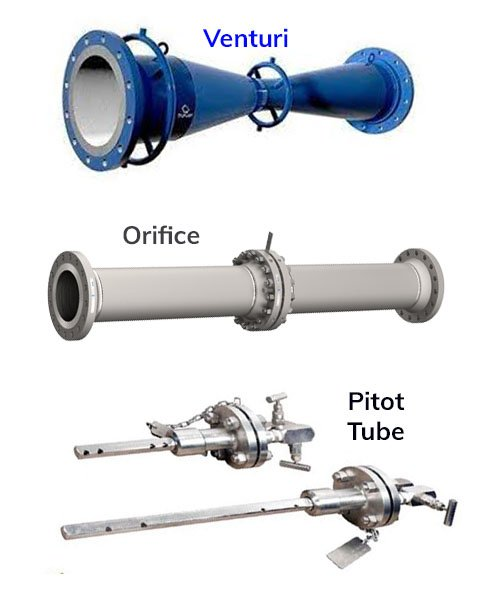You can check the video tutorial of the Vortex flow meter on the Endress+Hauser youtube channel.

### 3) Thermal Flow Meters

The thermal flowmeter uses the thermal properties of the fluid to measure the flow of fluid flowing through the pipe.

In a typical thermal flowmeter, a measured amount of heat is applied to the heater of the sensor. Some of this heat is lost to cool the fluid. As the flow of the fluid increased more amount of heat is lost. The thermal flow meter uses a temperature sensor to measure heat loss. The heat input and the amount of heat dissipated in the fluid is used to measure the flow.

You can check the video tutorial of the Vortex flow meter on the Endress+Hauser youtube channel.

• No need for temperature and pressure compensation.
• Wide range ratio, it can be up to 1:200
• Highly accurate and repeatable measurements
• Ability to measure low-pressure gas flow

Applications ⇒

• Measurement of flare gas flow
• Measuring emission from steam generators and boilers
• Measurement and recovery of land-filled gas
• Ethanol distillation and refining
• Compressed airflow

### 4) Vortex Flow Meters

Vortex flowmeter works on the principle of the vortex shedding principle, where oscillating vortexes occur when a fluid such water flow past a bluff body(non-streamed line object).

The frequency of vortex shedding is directly proportional to the mean flow velocity and thus to volume flow. It is ideal for the application where low maintenance cost is important.

You can check the video tutorial of the Vortex flow meter on the Endress+Hauser youtube channel.

• Suitable for measuring all objects like liquids, gases, and steam
• Mostly unaffected by changes in pressure, density, temperature and viscosity
• The high long term stability
• Wide temperature range

Applications ⇒

• Cooling Water
• Process Water
• Gas Flow where large turndown is required

### 5) Electromagnetic Flow Meters

Electromagnetic Flowmeter also called Mag Flowmeter works on the principle of FARADAY’S LAW OF INDUCTION. According to Faraday’s law voltage induced across any conductor when it passes through the magnetic field is directly proportional to the velocity of the medium, the density of the magnetic field, and the length of the conductor.

The representation in mathematical format is,

e = k * B * ν * d

where,

• e = Induced voltage
• k = constant
• B = Magnetic field strength
• ν = Velocity of the conductor
• d = length of the conductor

You can check the video tutorial of the Vortex flow meter on the Endress+Hauser youtube channel.

• There are no moving parts in the sensor, So suitable for wastewater application like mud-water, slurry water
• Not very much affected from upstream flow disturbances
• Mostly unaffected by changes in pressure, density, temperature and viscosity
• Meters can be used as bi-directional
• Capable of handling extremely low flow

Applications ⇒

• Mostly used in wastewater like mud-water or slurry water application
• High viscous fluid especially in food processing industries

### 6) Ultrasonic Flow Meters

An ultrasonic flow meter is defined as a meter that uses ultrasound waves to measure the volume of liquid flow. This is a volumetric flow meter. The construction of the ultrasonic flow meter consists of upstream and downstream transducers, reflectors, and sensor pipe.

It uses sound waves to analyze the volume of flow. At no flow condition, the frequencies of an ultrasonic wave transmitted into a pipe and its reflection from the fluid are the same. Under the flowing condition, the frequency of a reflected wave is different due to the Doppler effect. When a fluid flow from the pipe quickly its frequency shift increases linearly.

You can check the video tutorial of the Vortex flow meter on the Endress+Hauser youtube channel.

• High turndown
• Handle high pressure
• Handle extreme temperatures
• Low maintenance and highly reliable

Applications ⇒

• Natural gas and petroleum liquids
• Oil and Gas
• Water and wastewater
• Food and Beverages
• Pharmaceutical
• Metal and mining

### 7) Coriolis Mass Flow Meters

A Coriolis mass flowmeter work on the Coriolis effect is based on the principle of motion mechanics. As fluid moves through a vibrating tube, it is forced to accelerate as it moves toward the point of peak-amplitude vibration.

You can check the video tutorial of the Vortex flow meter on the Endress+Hauser youtube channel

## PLC Program to Calculate Flow of Gas

Here is my simple logic in the PLC program in ladder language to calculate the flow of gas flowing through a pipe.

Here I am using a venturi for the primary element to create a pressure difference and then use the differential pressure transmitter to calculate the pressure difference created by a venturi.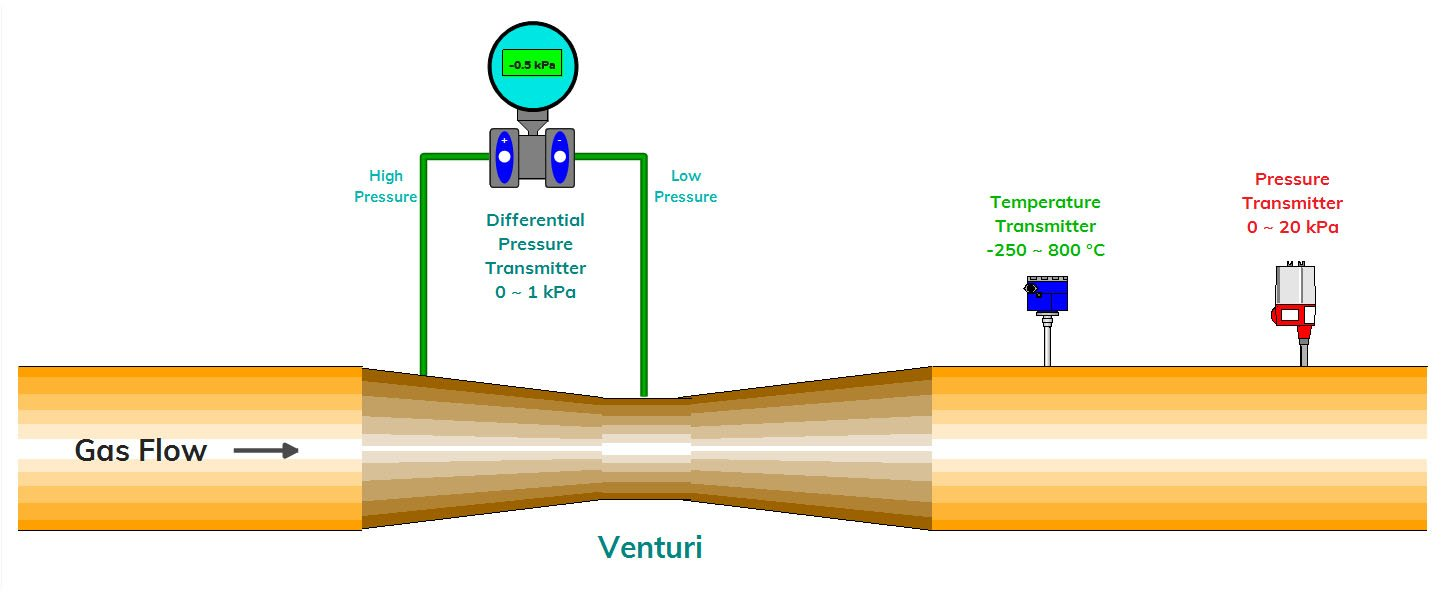Flow Measurement Example

The formula provided by the designer to calculate the flow (Nm3/hr) is,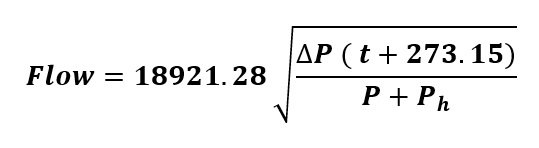Where,

• ΔP = Differential Pressure
• t = Temperature
• P = Line Pressure
• Ph = 99.805 kPa that is given as per the parameter of fluid, pipe length, etc.

The sensors I am using here is,

• DPT(Differential Pressure Transmitter, Range is 0 ~ 1 kPa)
• RTD(Temperature Transmitter, Range is -250 ~ 800 °C)
• Pressure, Range is 0 ~ 20 kPa

### Ladder Logic of Flow formula

The ladder logic of the flow formula is as given below,

• I am creating an “Always ON” and “Always OFF” bit in the network 1 and network 2. This is used in the scaling logic in the below network.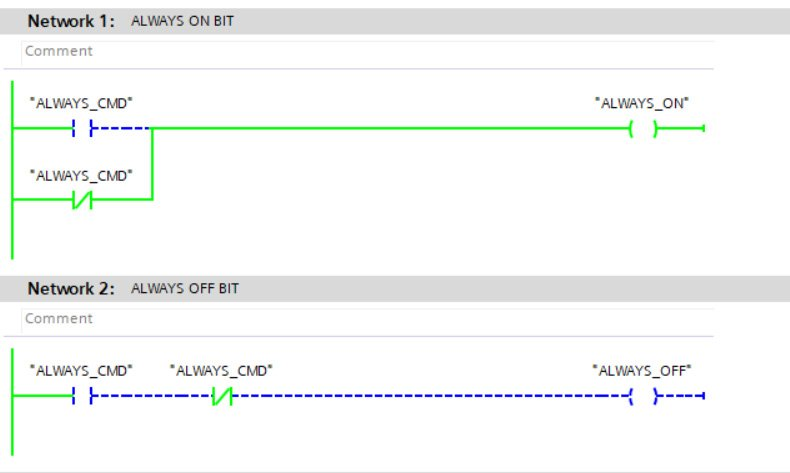• This network 3 represents the “SCALE” logic of the Pressure sensor. The range of the pressure transmitter is 0 to 20000 Pa.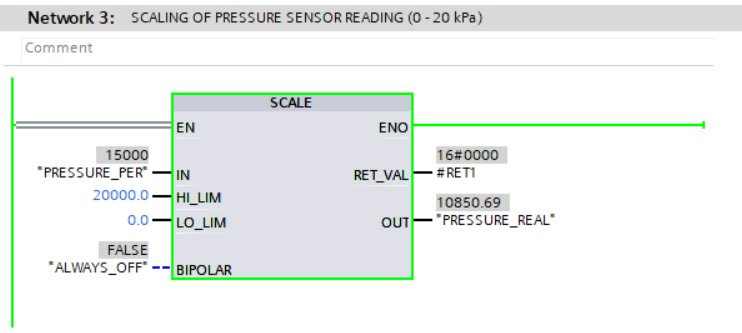• This network 4 represents the “SCALE” logic of the Differential Pressure sensor. The range of the differential pressure transmitter is 0 to 1000 Pa.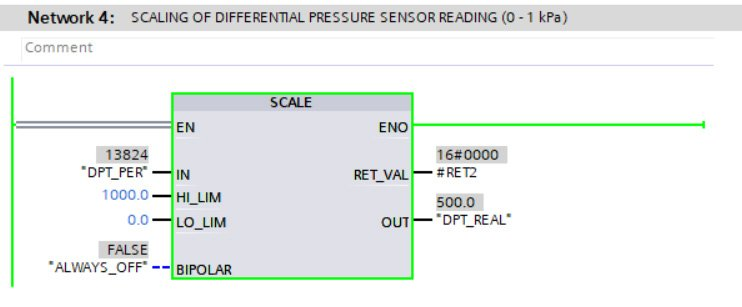• This network 5 represents the “SCALE” logic of the Temperature sensor. The range of the temperature transmitter is -200 to 850 ºC.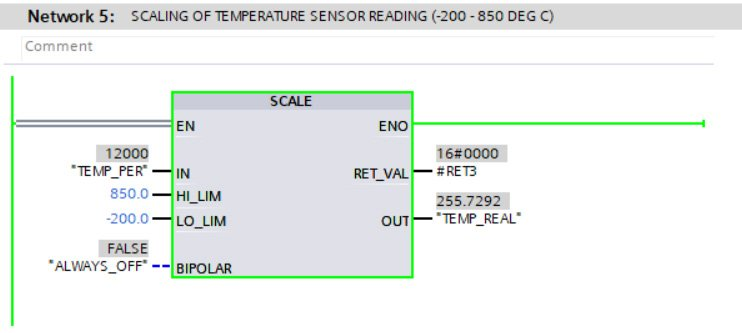• As per the formula, we are first adding 273.15 in the temperature and then multiply it with the differential pressure transmitter, then save it to Intermediate 2.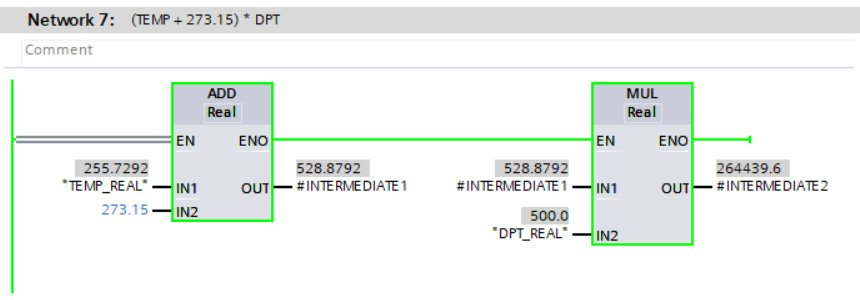• In this network 8, we are adding the pressure value with the maximum pressure value of 99805 Pa, and then save it to Intermediate 3.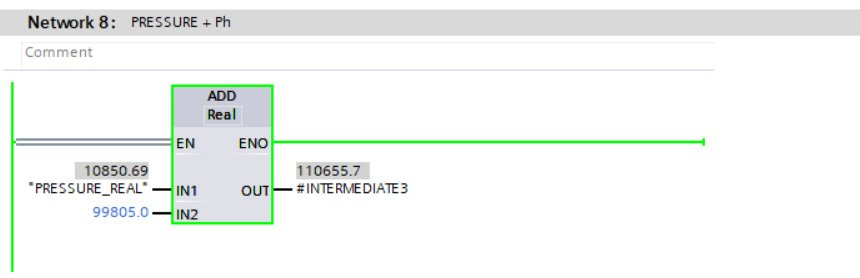• In this network 9, we are dividing Intermediate 2 by the Intermediate 3 and square root the value to Intermediate 5.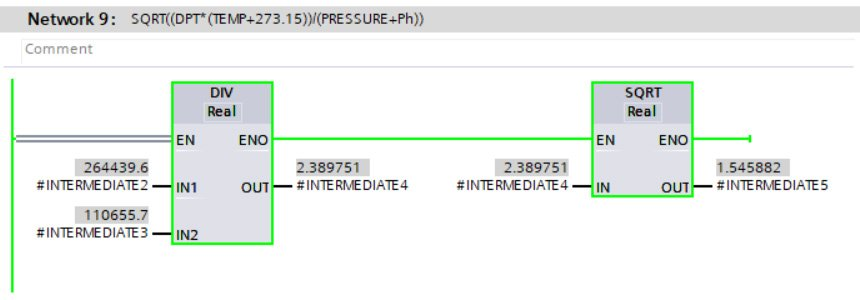• At last, in this network 10, we multiply the value of Intermediate 5 with constant value 18921.28. Finally, we get a flow here.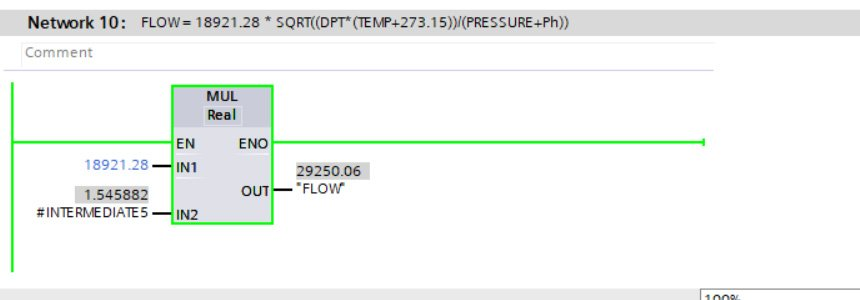I hope you like this blog, if you want more article like this then please comment down below. Thanks!

Here is the list of must-read articles about PLC and PLC programming,